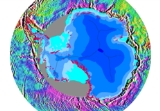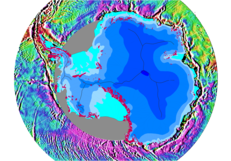xGravimetryEncyclopediaGravimetry is the measurement of the strength of a gravitational field. Gravimetry may be used when either the magnitude of gravitational field or the properties of matter responsible for its creation are of interest. The term gravimetry or gravimetric is also used in chemistry to define a class of analytical procedures, called gravimetric analysis
Gravimetric analysis
Gravimetric analysis describes a set of methods in analytical chemistry for the quantitative determination of an analyte based on the mass of a solid...

relying upon weighing a sample of material.

## Units of measurement

Gravity is usually measured in units of acceleration
Acceleration
In physics, acceleration is the rate of change of velocity with time. In one dimension, acceleration is the rate at which something speeds up or slows down. However, since velocity is a vector, acceleration describes the rate of change of both the magnitude and the direction of velocity. ...

. In the SI
Si
Si, si, or SI may refer to :- Measurement, mathematics and science :* International System of Units , the modern international standard version of the metric system...

system of units, the standard unit of acceleration is 1 metre per second squared
Metre per second squared
The metre per second squared is the unit of acceleration in the International System of Units . As a derived unit it is composed from the SI base units of length, the metre, and the standard unit of time, the second...

(abbreviated as m/s2). Other units include the gal (sometimes known as a galileo, in either case with symbol Gal), which equals 1 centimetre
Centimetre
A centimetre is a unit of length in the metric system, equal to one hundredth of a metre, which is the SI base unit of length. Centi is the SI prefix for a factor of . Hence a centimetre can be written as or — meaning or respectively...

per second squared, and the g
G-force
The g-force associated with an object is its acceleration relative to free-fall. This acceleration experienced by an object is due to the vector sum of non-gravitational forces acting on an object free to move. The accelerations that are not produced by gravity are termed proper accelerations, and...

(gn), equal to 9.80665 m/s2. The value of the gn approximately equals the acceleration due to gravity at the Earth's surface
Standard gravity
Standard gravity, or standard acceleration due to free fall, usually denoted by g0 or gn, is the nominal acceleration of an object in a vacuum near the surface of the Earth. It is defined as precisely , or about...

(although the actual acceleration g varies fractionally from place to place).

## How gravity is measured

An instrument used to measure gravity is known as a gravimeter
Gravimeter
A gravimeter or gravitometer is an instrument used in gravimetry for measuring the local gravitational field of the Earth. A gravimeter is a type of accelerometer, specialized for measuring the constant downward acceleration of gravity, which varies by about 0.5% over the surface of the Earth...

, or gravitometer. Since general relativity
General relativity
General relativity or the general theory of relativity is the geometric theory of gravitation published by Albert Einstein in 1916. It is the current description of gravitation in modern physics...

regards the effects of gravity as indistinguishable from the effects of acceleration
Acceleration
In physics, acceleration is the rate of change of velocity with time. In one dimension, acceleration is the rate at which something speeds up or slows down. However, since velocity is a vector, acceleration describes the rate of change of both the magnitude and the direction of velocity. ...

, gravimeters may be regarded as special purpose accelerometer
Accelerometer
An accelerometer is a device that measures proper acceleration, also called the four-acceleration. This is not necessarily the same as the coordinate acceleration , but is rather the type of acceleration associated with the phenomenon of weight experienced by a test mass that resides in the frame...

s. Many weighing scale
Weighing scale
A weighing scale is a measuring instrument for determining the weight or mass of an object. A spring scale measures weight by the distance a spring deflects under its load...

s may be regarded as simple gravimeters. In one common form, a spring
Spring (device)
A spring is an elastic object used to store mechanical energy. Springs are usually made out of spring steel. Small springs can be wound from pre-hardened stock, while larger ones are made from annealed steel and hardened after fabrication...

is used to counteract the force of gravity pulling on an object. The change in length of the spring may be calibrated to the force required to balance the gravitational pull. The resulting measurement may be made in units of force (such as the newton), but is more commonly made in units of gal
Gal (unit)
The gal, sometimes called galileo, is a unit of acceleration used extensively in the science of gravimetry. The gal is defined as 1 centimeter per second squared ....

s.

More sophisticated gravimeters are used when precise measurements are needed. When measuring the Earth's gravitational field
Earth's gravity
The gravity of Earth, denoted g, refers to the acceleration that the Earth imparts to objects on or near its surface. In SI units this acceleration is measured in metres per second per second or equivalently in newtons per kilogram...

, measurements are made to the precision of microgals to find density variations in the rocks making up the Earth. Several types of gravimeters exist for making these measurements, including some that are essentially refined versions of the spring scale described above. These measurements are used to define gravity anomalies
Gravity anomaly
A gravity anomaly is the difference between the observed acceleration of Earth's gravity and a value predicted from a model.-Geodesy and geophysics:...

.

Besides precision
Accuracy and precision
In the fields of science, engineering, industry and statistics, the accuracy of a measurement system is the degree of closeness of measurements of a quantity to that quantity's actual value. The precision of a measurement system, also called reproducibility or repeatability, is the degree to which...

, also stability is an important property of a gravimeter, as it allows the monitoring of gravity changes. These changes can be the result of mass displacements inside the Earth, or of vertical movements of the Earth's crust on which measurements are being made: remember that gravity decreases 0.3 mGal for every metre of height
Height
Height is the measurement of vertical distance, but has two meanings in common use. It can either indicate how "tall" something is, or how "high up" it is. For example "The height of the building is 50 m" or "The height of the airplane is 10,000 m"...

. The study of gravity changes belongs to geodynamics
Geodynamics
Geodynamics is a subfield of geophysics dealing with dynamics of the Earth. It applies physics, chemistry and mathematics to the understanding of how mantle convection leads to plate tectonics and geologic phenomena such as seafloor spreading, mountain building, volcanoes, earthquakes, faulting and...

.

The majority of modern gravimeters use specially-designed metal or quartz
Quartz
Quartz is the second-most-abundant mineral in the Earth's continental crust, after feldspar. It is made up of a continuous framework of SiO4 silicon–oxygen tetrahedra, with each oxygen being shared between two tetrahedra, giving an overall formula SiO2. There are many different varieties of quartz,...

zero-length springs to support the test mass. Zero length springs do not follow Hooke's Law
Hooke's law
In mechanics, and physics, Hooke's law of elasticity is an approximation that states that the extension of a spring is in direct proportion with the load applied to it. Many materials obey this law as long as the load does not exceed the material's elastic limit. Materials for which Hooke's law...

, instead they have a force proportional to their length. The special property of these springs is that the natural resonant period of oscillation
Oscillation
Oscillation is the repetitive variation, typically in time, of some measure about a central value or between two or more different states. Familiar examples include a swinging pendulum and AC power. The term vibration is sometimes used more narrowly to mean a mechanical oscillation but sometimes...

of the spring-mass system can be made very long - approaching a thousand seconds. This detunes the test mass from most local vibration and mechanical noise
Noise (acoustic)
Acoustic noise is any sound in the acoustic domain, either deliberate or unintended. It is important to recognise that the term "noise" is also used to refer to other, non-audible forms, especially in electronics and in the radio/radar spectrum.-Environmental noise:Environmental noise is the...

, increasing the sensitivity and utility of the gravimeter. Quartz and metal springs are chosen for different reasons; quartz springs are less affected by magnetic and electric fields while metal springs have a much lower drift (elongation) with time. The test mass is sealed in an air-tight container so that tiny changes of barometric pressure from blowing wind and other weather do not change the buoyancy of the test mass in air.

Spring gravimeters are, in practice, relative instruments which measure the difference in gravity between different locations. A relative instrument also requires calibration by
comparing instrument readings taken at locations with known complete or absolute values of gravity. Absolute gravimeters provide such measurements by determining the gravitational acceleration of a test mass in vacuum. A test mass is allowed to fall freely inside a vacuum chamber and its position is measured with a laser interferometer and timed with an atomic
clock. The laser wavelength is known to ±0.025 ppb
PPB
PPB can stand for:* Party political broadcast, a type of political programming in the United Kingdom* parts-per-billion, a unit of concentration* Portland Police Bureau, a police agency for the city of Portland...

and the clock is stable to ±0.03 ppb
as well. Great care must be taken to minimize the effects of perturbing forces such as
residual air resistance (even in vacuum), vibration, and magnetic forces. Such instruments are capable of an accuracy of about two parts per billion or 0.002 mGal and reference their measurement to atomic standards of length and time. Their primary use is for calibrating relative instruments, monitoring crustal deformation, and in geophysical studies requiring high accuracy and stability. However, absolute instruments are somewhat larger and significantly more expensive
than relative spring gravimeters, and are thus relatively rare.

Gravimeters have been designed to mount in vehicles, including aircraft, ships and submarines. These special gravimeters isolate acceleration from the movement of the vehicle, and subtract it from measurements. The acceleration of the vehicles is often hundreds or thousands of times stronger than the changes being measured. A gravimeter (the Lunar Surface Gravimeter) was also deployed on the surface of the moon during the Apollo 17 mission, but did not work due to a design error. A second device (the Traverse Gravimeter Experiment) functioned as anticipated.

## Microgravimetry

Microgravimetry is a rising and important branch developed on the foundation of classical gravimetry. Microgravity investigations are carried out in order to solve various problems of engineering geology, mainly location of voids and their monitoring. Very detailed measurements of high accuracy can indicate voids of any origin, provided the size and depth are large enough to produce gravity effect stronger than is the level of confidence of relevant gravity signal.

## History

The modern gravimeter was developed by Lucien LaCoste
Lucien LaCoste
Lucien LaCoste was a prominent physicist, metrologist. He was coinventor of the modern gravimeter, invented the zero-length spring, and vehicle-mounted gravimeters. He was also co-founder of a prominent company selling gravimetric instruments....

and Arnold Romberg in 1936.

They also invented most subsequent refinements, including the ship-mounted gravimeter, in 1965, temperature-resistant instruments for deep boreholes, and lightweight hand-carried instruments. Most of their designs remain in use (2005) with refinements in data collection and data processing.